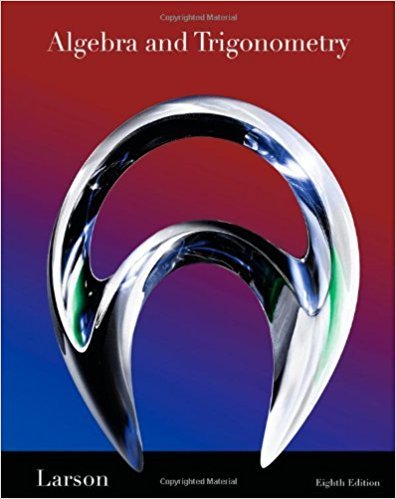×
×

# Solutions for Chapter 10.1: Matrices and Systems of Equations## Full solutions for Algebra and Trigonometry | 8th Edition

ISBN: 9781439048474Solutions for Chapter 10.1: Matrices and Systems of Equations

Solutions for Chapter 10.1
4 5 0 370 Reviews
15
4
##### ISBN: 9781439048474

Chapter 10.1: Matrices and Systems of Equations includes 123 full step-by-step solutions. Algebra and Trigonometry was written by and is associated to the ISBN: 9781439048474. This textbook survival guide was created for the textbook: Algebra and Trigonometry, edition: 8. This expansive textbook survival guide covers the following chapters and their solutions. Since 123 problems in chapter 10.1: Matrices and Systems of Equations have been answered, more than 51089 students have viewed full step-by-step solutions from this chapter.

Key Math Terms and definitions covered in this textbook
• Associative Law (AB)C = A(BC).

Parentheses can be removed to leave ABC.

• Complete solution x = x p + Xn to Ax = b.

(Particular x p) + (x n in nullspace).

• Elimination.

A sequence of row operations that reduces A to an upper triangular U or to the reduced form R = rref(A). Then A = LU with multipliers eO in L, or P A = L U with row exchanges in P, or E A = R with an invertible E.

• Fibonacci numbers

0,1,1,2,3,5, ... satisfy Fn = Fn-l + Fn- 2 = (A7 -A~)I()q -A2). Growth rate Al = (1 + .J5) 12 is the largest eigenvalue of the Fibonacci matrix [ } A].

• Full row rank r = m.

Independent rows, at least one solution to Ax = b, column space is all of Rm. Full rank means full column rank or full row rank.

• Kronecker product (tensor product) A ® B.

Blocks aij B, eigenvalues Ap(A)Aq(B).

• Krylov subspace Kj(A, b).

The subspace spanned by b, Ab, ... , Aj-Ib. Numerical methods approximate A -I b by x j with residual b - Ax j in this subspace. A good basis for K j requires only multiplication by A at each step.

• Linearly dependent VI, ... , Vn.

A combination other than all Ci = 0 gives L Ci Vi = O.

• Network.

A directed graph that has constants Cl, ... , Cm associated with the edges.

• Normal equation AT Ax = ATb.

Gives the least squares solution to Ax = b if A has full rank n (independent columns). The equation says that (columns of A)·(b - Ax) = o.

• Nullspace N (A)

= All solutions to Ax = O. Dimension n - r = (# columns) - rank.

• Projection matrix P onto subspace S.

Projection p = P b is the closest point to b in S, error e = b - Pb is perpendicularto S. p 2 = P = pT, eigenvalues are 1 or 0, eigenvectors are in S or S...L. If columns of A = basis for S then P = A (AT A) -1 AT.

• Reflection matrix (Householder) Q = I -2uuT.

Unit vector u is reflected to Qu = -u. All x intheplanemirroruTx = o have Qx = x. Notice QT = Q-1 = Q.

• Row picture of Ax = b.

Each equation gives a plane in Rn; the planes intersect at x.

• Saddle point of I(x}, ... ,xn ).

A point where the first derivatives of I are zero and the second derivative matrix (a2 II aXi ax j = Hessian matrix) is indefinite.

• Schwarz inequality

Iv·wl < IIvll IIwll.Then IvTAwl2 < (vT Av)(wT Aw) for pos def A.

• Subspace S of V.

Any vector space inside V, including V and Z = {zero vector only}.

• Trace of A

= sum of diagonal entries = sum of eigenvalues of A. Tr AB = Tr BA.

• Transpose matrix AT.

Entries AL = Ajj. AT is n by In, AT A is square, symmetric, positive semidefinite. The transposes of AB and A-I are BT AT and (AT)-I.

• Triangle inequality II u + v II < II u II + II v II.

For matrix norms II A + B II < II A II + II B II·

×